Distribution & Network Models Chapter Exam

Exam Instructions:

Choose your answers to the questions and click 'Next' to see the next set of questions. You can skip questions if you would like and come back to them later with the yellow "Go To First Skipped Question" button. When you have completed the practice exam, a green submit button will appear. Click it to see your results. Good luck!

Page 1

Question 1 1. Brown's Business is using the Minimum Cell Cost method to solve an unbalanced transportation problem. Using the following matrix, what is the total cost for transportation?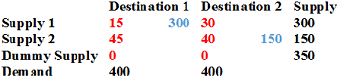Question 3 3. This image represents which of the following?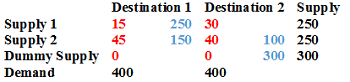Page 2

Question 6 6. Given the following information, which route has the least cost for transportation?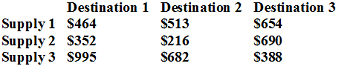Question 7 7. Given the following information where red is the cost and blue is the supply, calculate the total transportation costs.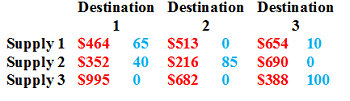Page 5

Question 22 22. The original matrix in an assignment problem and the end matrix after performing the Hungarian Algorithm are shown. Based on this, what is the TOTAL minimum cost of the problem?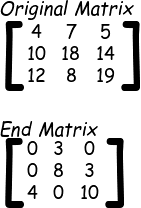Question 23 23. Suppose you are using the Hungarian Algorithm for an assignment problem, and you performed the first two steps of the algorithm (subtracting row minima and subtracting column minima). If the matrix shown is the result, which of the following statements is true?Question 25 25. Suppose you are using the Hungarian Algorithm on the matrix shown. Which of the following matrices would be the result after subtracting row minima AND subtracting column minima? In other words, what matrix would we have after performing the first two steps of the Hungarian Algorithm?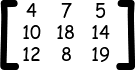Distribution & Network Models Chapter Exam Instructions

Choose your answers to the questions and click 'Next' to see the next set of questions. You can skip questions if you would like and come back to them later with the yellow "Go To First Skipped Question" button. When you have completed the practice exam, a green submit button will appear. Click it to see your results. Good luck!

Support